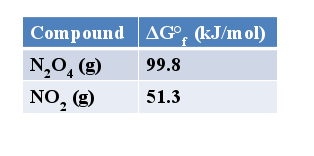# Problem: Calculate the equilibrium constant, K, for the reaction at 298 K: N2O4 (g) ⇌  2 NO2 (g)

###### FREE Expert Solution
91% (107 ratings)
###### Problem Details

Calculate the equilibrium constant, K, for the reaction at 298 K:

N2O4 (g) ⇌  2 NO2 (g)What scientific concept do you need to know in order to solve this problem?

Our tutors have indicated that to solve this problem you will need to apply the Gibbs Free Energy concept. You can view video lessons to learn Gibbs Free Energy. Or if you need more Gibbs Free Energy practice, you can also practice Gibbs Free Energy practice problems.

What is the difficulty of this problem?

Our tutors rated the difficulty ofCalculate the equilibrium constant, K, for the reaction at 2...as low difficulty.

How long does this problem take to solve?

Our expert Chemistry tutor, Jules took 2 minutes and 15 seconds to solve this problem. You can follow their steps in the video explanation above.

What professor is this problem relevant for?

Based on our data, we think this problem is relevant for Professor Davis' class at UCF.